# 11.5 Counting principles  (Page 2/12)

 Page 2 / 12

## The multiplication principle

According to the Multiplication Principle    , if one event can occur in $m$ ways and a second event can occur in $n$ ways after the first event has occurred, then the two events can occur in $m×n$ ways. This is also known as the Fundamental Counting Principle    .

## Using the multiplication principle

Diane packed 2 skirts, 4 blouses, and a sweater for her business trip. She will need to choose a skirt and a blouse for each outfit and decide whether to wear the sweater. Use the Multiplication Principle to find the total number of possible outfits.

To find the total number of outfits, find the product of the number of skirt options, the number of blouse options, and the number of sweater options.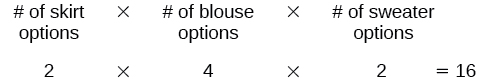There are 16 possible outfits.

A restaurant offers a breakfast special that includes a breakfast sandwich, a side dish, and a beverage. There are 3 types of breakfast sandwiches, 4 side dish options, and 5 beverage choices. Find the total number of possible breakfast specials.

There are 60 possible breakfast specials.

## Finding the number of permutations of n Distinct objects

The Multiplication Principle can be used to solve a variety of problem types. One type of problem involves placing objects in order. We arrange letters into words and digits into numbers, line up for photographs, decorate rooms, and more. An ordering of objects is called a permutation    .

## Finding the number of permutations of n Distinct objects using the multiplication principle

To solve permutation problems, it is often helpful to draw line segments for each option. That enables us to determine the number of each option so we can multiply. For instance, suppose we have four paintings, and we want to find the number of ways we can hang three of the paintings in order on the wall. We can draw three lines to represent the three places on the wall.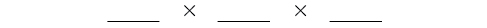There are four options for the first place, so we write a 4 on the first line.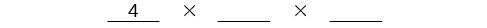After the first place has been filled, there are three options for the second place so we write a 3 on the second line.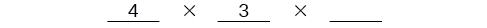After the second place has been filled, there are two options for the third place so we write a 2 on the third line. Finally, we find the product.There are 24 possible permutations of the paintings.

Given $\text{\hspace{0.17em}}n\text{\hspace{0.17em}}$ distinct options, determine how many permutations there are.

1. Determine how many options there are for the first situation.
2. Determine how many options are left for the second situation.
3. Continue until all of the spots are filled.
4. Multiply the numbers together.

## Finding the number of permutations using the multiplication principle

At a swimming competition, nine swimmers compete in a race.

1. How many ways can they place first, second, and third?
2. How many ways can they place first, second, and third if a swimmer named Ariel wins first place? (Assume there is only one contestant named Ariel.)
3. How many ways can all nine swimmers line up for a photo?
1. Draw lines for each place.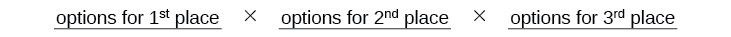There are 9 options for first place. Once someone has won first place, there are 8 remaining options for second place. Once first and second place have been won, there are 7 remaining options for third place.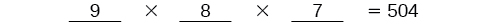Multiply to find that there are 504 ways for the swimmers to place.

2. Draw lines for describing each place.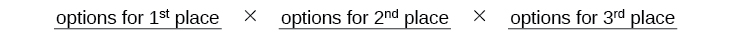We know Ariel must win first place, so there is only 1 option for first place. There are 8 remaining options for second place, and then 7 remaining options for third place.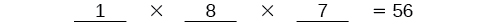Multiply to find that there are 56 ways for the swimmers to place if Ariel wins first.

3. Draw lines for describing each place in the photo.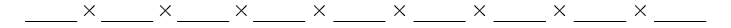There are 9 choices for the first spot, then 8 for the second, 7 for the third, 6 for the fourth, and so on until only 1 person remains for the last spot.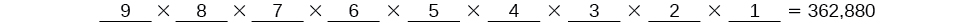There are 362,880 possible permutations for the swimmers to line up.

can you not take the square root of a negative number
Suppose P= {-3,1,3} Q={-3,-2-1} and R= {-2,2,3}.what is the intersection
can I get some pretty basic questions
In what way does set notation relate to function notation
Ama
is precalculus needed to take caculus
It depends on what you already know. Just test yourself with some precalculus questions. If you find them easy, you're good to go.
Spiro
the solution doesn't seem right for this problem
what is the domain of f(x)=x-4/x^2-2x-15 then
x is different from -5&3
Seid
All real x except 5 and - 3
Spiro
how to prroved cos⁴x-sin⁴x= cos²x-sin²x are equal
Don't think that you can.
Elliott
how do you provided cos⁴x-sin⁴x = cos²x-sin²x are equal
What are the question marks for?
Elliott
Someone should please solve it for me Add 2over ×+3 +y-4 over 5 simplify (×+a)with square root of two -×root 2 all over a multiply 1over ×-y{(×-y)(×+y)} over ×y
For the first question, I got (3y-2)/15 Second one, I got Root 2 Third one, I got 1/(y to the fourth power) I dont if it's right cause I can barely understand the question.
Is under distribute property, inverse function, algebra and addition and multiplication function; so is a combined question
Abena
find the equation of the line if m=3, and b=-2
graph the following linear equation using intercepts method. 2x+y=4
Ashley
how
Wargod
what?
John
ok, one moment
UriEl
how do I post your graph for you?
UriEl
it won't let me send an image?
UriEl
also for the first one... y=mx+b so.... y=3x-2
UriEl
y=mx+b you were already given the 'm' and 'b'. so.. y=3x-2
Tommy
Please were did you get y=mx+b from
Abena
y=mx+b is the formula of a straight line. where m = the slope & b = where the line crosses the y-axis. In this case, being that the "m" and "b", are given, all you have to do is plug them into the formula to complete the equation.
Tommy
thanks Tommy
Nimo
0=3x-2 2=3x x=3/2 then . y=3/2X-2 I think
Given
co ordinates for x x=0,(-2,0) x=1,(1,1) x=2,(2,4)
neil
"7"has an open circle and "10"has a filled in circle who can I have a set builder notation
Where do the rays point?
Spiro
x=-b+_Гb2-(4ac) ______________ 2a
I've run into this: x = r*cos(angle1 + angle2) Which expands to: x = r(cos(angle1)*cos(angle2) - sin(angle1)*sin(angle2)) The r value confuses me here, because distributing it makes: (r*cos(angle2))(cos(angle1) - (r*sin(angle2))(sin(angle1)) How does this make sense? Why does the r distribute once
so good
abdikarin
this is an identity when 2 adding two angles within a cosine. it's called the cosine sum formula. there is also a different formula when cosine has an angle minus another angle it's called the sum and difference formulas and they are under any list of trig identities
strategies to form the general term
carlmark
consider r(a+b) = ra + rb. The a and b are the trig identity.
Mike
How can you tell what type of parent function a graph is ?
generally by how the graph looks and understanding what the base parent functions look like and perform on a graph
William
if you have a graphed line, you can have an idea by how the directions of the line turns, i.e. negative, positive, zero
William
y=x will obviously be a straight line with a zero slope
William
y=x^2 will have a parabolic line opening to positive infinity on both sides of the y axis vice versa with y=-x^2 you'll have both ends of the parabolic line pointing downward heading to negative infinity on both sides of the y axis
William
y=x will be a straight line, but it will have a slope of one. Remember, if y=1 then x=1, so for every unit you rise you move over positively one unit. To get a straight line with a slope of 0, set y=1 or any integer.
Aaron
yes, correction on my end, I meant slope of 1 instead of slope of 0
William
what is f(x)=
I don't understand
Joe
Typically a function 'f' will take 'x' as input, and produce 'y' as output. As 'f(x)=y'. According to Google, "The range of a function is the complete set of all possible resulting values of the dependent variable (y, usually), after we have substituted the domain."
Thomas
Sorry, I don't know where the "Â"s came from. They shouldn't be there. Just ignore them. :-)
Thomas
Darius
Thanks.
Thomas
Â
Thomas
It is the Â that should not be there. It doesn't seem to show if encloses in quotation marks. "Â" or 'Â' ... Â
Thomas
Now it shows, go figure?
ThomasByByBy RhodesBy Naveen TomarByBy RhodesBy Jordon Humphreys